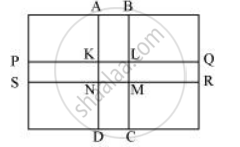Share

# Through a Rectangular Field of Length 90 M and Breadth 60 M, Two Roads Are Constructed Which Are Parallel to the Sides and Cut Each Other at Right Angles Through the Centre of the Fields. If the Width of Each Road is 3 M, Find - CBSE Class 7 - Mathematics

ConceptConcept of Application of Conversion

#### Question

Through a rectangular field of length 90 m and breadth 60 m, two roads are constructed which are parallel to the sides and cut each other at right angles through the centre of the fields. If the width of each road is 3 m, find

1)the area covered by the roads.

2) the cost of constructing the roads at the rate of Rs 110 per m2.

#### SolutionLength (l) of field = 90 m

Breadth (b) of field = 60 m

Area of field = 90 × 60 = 5400 m2

Length of road PQRS = 90 m

Length of road ABCD = 60 m

Width of each road = 3 m

Area of the roads = ar (PQRS) + ar (ABCD) − ar (KLMN)

= (90 × 3) + (60 × 3) − (3 × 3)

= 270 + 180 − 9 = 441 m2

Cost for constructing 1 m2 road = Rs 110

Cost for constructing 441 m2 road = 110 × 441 = Rs 48510

Is there an error in this question or solution?

#### APPEARS IN

NCERT Solution for Mathematics for Class 7 (2018 to Current)
Chapter 11: Perimeter and Area
Ex. 11.40 | Q: 7 | Page no. 227
Solution Through a Rectangular Field of Length 90 M and Breadth 60 M, Two Roads Are Constructed Which Are Parallel to the Sides and Cut Each Other at Right Angles Through the Centre of the Fields. If the Width of Each Road is 3 M, Find Concept: Concept of Application of Conversion.
S